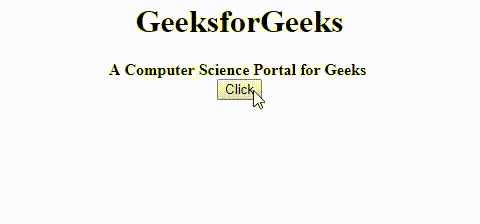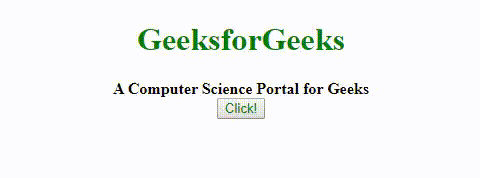GFG App
Open AppBrowser
Continue

# How to call functions after previous function is completed in jQuery ?

The task is to execute a series of functions sequentially in JavaScript. That is, execute function two ONLY after the first function has completed its execution.

Syntax:

`functionName();`

Approach: This problem can be solved in multiple ways.

• We can fix (hard-code) a callback function at the end of function one. Doing this makes sure that the callback function is always called when the function finishes its execution. However, a problem with this approach is that we can’t change the callback function later at runtime. We will have to either remove that line of code OR modify it.
• Another way is to pass the callback function as a parameter to function one and make function one call this new callback function at the end of its life. This approach is more flexible as it allows us to change the callback function at runtime without actually modifying any lines of code.

Let’s take a look at each of them in detail.

Example 1: In this example, we will fix (hard-code) a callback function at the end of function one.

## html

 `` `<``html``>`   `<``head``>` `    ``<``title``>Call a function after completing a function` ``   `<``body``>` `    ``<``center``>` `        ``<``h1` `style``=``"color: green;"``>GeeksforGeeks` `        ``<``b``>A Computer Science Portal for Geeks<``br``>` `        ``<``button` `style``=``"color: green;"``>Click!` `        ``<``p``>` `        ``<``script` `src``=` `"https://code.jquery.com/jquery-3.4.1.min.js"``>` `        ``` `        ``<``script` `type``=``"text/javascript"``>` `            ``\$('button').click(function () {` `                ``console.log("Button clicked!");`   `                ``// Execute callbackSecond at the end` `                ``// of its execution` `                ``callbackFirst(callbackSecond);` `            ``});`   `            ``function callbackFirst(callbackFn) {` `                ``console.log("First Function start");` `                ``for (var i = 1; i < ``10``; i++) {` `                    ``\$('p').append(i + " ");` `                ``}` `                ``console.log("First Function end");`   `                ``/* Execute callbackFn() now as its` `                ``the end of callbackFirst() This ` `                ``function was passed as a parameter */` `                ``callbackFn();` `            ``}`   `            ``function callbackSecond() {` `                ``console.log("Second Function start");` `                ``\$('body').css({` `                    ``'background-color': 'green'` `                ``});` `                ``\$('h1').css({` `                    ``'color': 'black'` `                ``});` `                ``console.log("Second Function end");` `            ``}` `        ``` `    ``` `` ``

Output:In the console after clicking button:

```Button clicked!
First Function start
First Function end
Second Function start
Second Function end```

Example 2: In this example, we will pass a callback function as a parameter to the function one so that it can call this new callback function at the end of its execution. The task is to execute the callbackFirst function first and then execute the callbackSecond function. Passing callbackSecond as a parameter to the callback first.

## HTML

 `` `<``html``>`   `<``head``>` `    ``<``title``>Call a function after completing a function` ``   `<``body``>` `    ``<``center``>` `        ``<``h1` `style``=``"color: green;"``>GeeksforGeeks` `        ``<``b``>A Computer Science Portal for Geeks<``br``>` `        ``<``button` `style``=``"color: green;"``>Click!` `        ``<``p``>` `        ``<``script` `src``=` `"https://code.jquery.com/jquery-3.4.1.min.js"``>` `        ``` `        ``<``script` `type``=``"text/javascript"``>` `            ``\$('button').click(function () {` `                ``console.log("Button clicked!");`   `                ``// Execute callbackSecond at the end` `                ``// of its execution` `                ``callbackFirst(callbackSecond);` `            ``});`   `            ``function callbackFirst(callbackFn) {` `                ``console.log("First Function start");` `                ``for (var i = 1; i < ``10``; i++) {` `                    ``\$('p').append(i + " ");` `                ``}` `                ``console.log("First Function end");`   `                ``/* Execute callbackFn() now as its` `                ``the end of callbackFirst() This ` `                ``function was passed as a parameter */` `                ``callbackFn();` `            ``}`   `            ``function callbackSecond() {` `                ``console.log("Second Function start");` `                ``\$('body').css({` `                    ``'background-color': 'green'` `                ``});` `                ``\$('h1').css({` `                    ``'color': 'black'` `                ``});` `                ``console.log("Second Function end");` `            ``}` `        ``` `    ``` `` ``

Output:In the console after clicking button:

```Button clicked!
First Function start
First Function end
Second Function start
Second Function end```

jQuery is an open-source JavaScript library that simplifies the interactions between an HTML/CSS document, It is widely famous for its philosophy of “Write less, do more”. You can learn jQuery from the ground up by following this jQuery Tutorial and jQuery Examples.

My Personal Notes arrow_drop_up